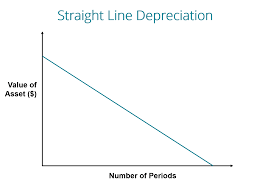## How to Calculate and Solve for Depreciation Value | Straight line Method | DepreciationThe image above represents depreciation value.

To compute for depreciation value, three essential value are needed and these parameters are Present Amount or Worth (P), Salvage Value (S) and Total Estimated Life of the Asset (N).

The formula for calculating depreciation value:

D = (P – S) / N

Where:

P = Present worth or amount
N = Total estimated life of an asset
S = Salvage value

Let’s solve an example;
Find the depreciation value when the present amount or worth is 9, the salvage value is 4 and the total estimated life of an asset is 10.

This implies that;

P = Present worth or amount = 9
N = Total estimated life of an asset = 10
S = Salvage value = 4

D = (P – S) / N
D = (9 – 4) / 10
D = 5 / 10
D = 0.5

Therefore, the depreciation value is ₦0.5.

Calculating the Present Amount or Worth when the Depreciation Value, the Total Estimated life of an Asset and the Salvage Value is Given.

P = (D x N) + S

Where;

P = Present worth or amount
D = Depreciation Value
N = Total estimated life of an asset
S = Salvage value

Let’s solve an example;
Find the present amount or worth when the depreciation value is 22, the total estimated life of an asset is 12 and the salvage value is 8.

This implies that;

D = Depreciation Value = 22
N = Total estimated life of an asset = 12
S = Salvage value = 8

P = (D x N) + S
P = (22 x 12) + 8
P = 264 + 8
P = 272

Therefore, the present amount or worth is 272.